• +91 9971497814
• info@interviewmaterial.com

# RD Chapter 12- Heron-s Formula Ex-VSAQS Interview Questions Answers

### Related Subjects

Question 1 : In two congruent triangles ABC and DEF, if AB = DE and BC = EF. Name the pairs of equal angles.

Answer 1 :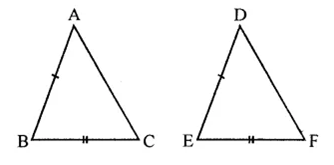∆ABC ≅ ∆DEF
and AB = DE, BC = EF
∴ ∠A = ∠D, ∠B = ∠E and ∠C = ∠F

Question 2 : In two triangles ABC and DEF, it is given that ∠A = ∠D, ∠B = ∠E and ∠C = ∠F. Are the two triangles necessarily congruent?No, as the triangles are equiangular, so similar.

Question 3 : If ABC and DEF are two triangles such that AC = 2.5 cm, BC = 5 cm, ∠C = 75°, DE = 2.5 cm, DF = 5 cm and ∠D = 75°. Are two triangles congruent?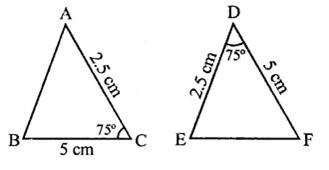Answer 3 : Yes, triangles are congruent (SAS axiom)

Question 4 : In two triangles ABC and ADC, if AB = AD and BC = CD. Are they congruent?

Yes, these are congruent
In two triangles ABC are ADC,
BC = CD (Given)
and AC = AC (Common)
∴ ∆sABC ≅ AADC (SSS axiom)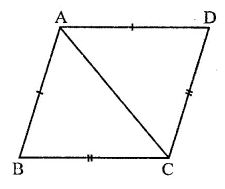Question 5 : In triangles ABC and CDE, if AC = CE, BC = CD, ∠A = 60°, ∠C – 30° and ∠D = 90°. Are two triangles congruent?

Yes, triangles are congruent because,
In ∆ABC, and ∆CDE,
AC = CE
BC = CD ∠C = 30°
∴ ∆ABC ≅ ∆CDE (SAS axiom)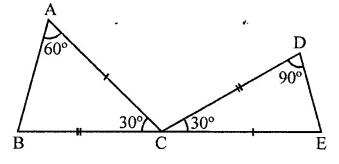Question 6 : ABC is an isosceles triangle in which AB = AC. BE and CF are its two medians. Show that BE = CF.

Given : In ∆ABC, AB = AC
BE and CF are two medians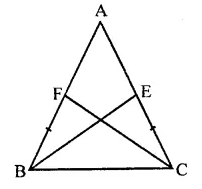To prove : BE = CF
Proof: In ∆ABE and ∆ACF.
AB = AC (Given)
∠A = ∠A (Common)
AE = AF (Half of equal sides)
∴ ∆ABE ≅ ∆ACF (SAS axiom)
∴ BE = CF (c.p.c.t.)

Question 7 : Find the measure of each angle of an equilateral triangle.

In ∆ABC,
AB = AC = BC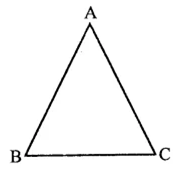∵ AB = AC
∴ ∠C = ∠B …(i)
(Angles opposite to equal sides)
Similarly,
AC = BC
∴ ∠B = ∠A …(ii)
From (i) and (ii),
∠A = ∠B = ∠C
But ∠A + ∠B + ∠C = 180°
(Sum of angles of a triangle)
∴ ∠A + ∠B + ∠C =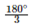= 60°

Question 8 : CDE is an equilateral triangle formed on a side CD of a square ABCD. Show that ∆ADE ≅ ∆BCE.

Given : An equilateral ACDE is formed on the side of square ABCD. AE and BE are joined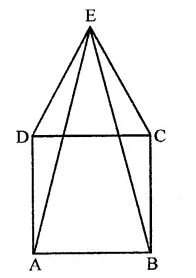To prove : ∆ADE ≅ ∆BCE
Proof : In ∆ADE and ∆BCE,
AD = BC (Sides of a square)
DE = CE (Sides of equilateral triangle)
∠ADE = ∠BCE(Each = 90° + 60° = 150°)
∴ AADE ≅ ABCE (SAS axiom)

Question 9 : Prove that the sum of three altitude of a triangle is less than the sum of its sides.

Given : In ∆ABC, AD, BE and CF are the altitude of ∆ABC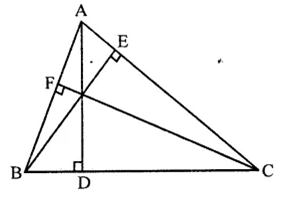To prove : AD + BE + CF < AB + BC + CA
Proof : In right ∆ABD, ∠D = 90°
Then other two angles are acute
∵ ∠B < ∠D
Similarly, in ∆BEC and ∆ABE we can prove thatBE and CF < CA …(iii)
AD + BE -t CF < AB + BC + CA

Question 10 : In the figure, if AB = AC and ∠B = ∠C. Prove that BQ = CP.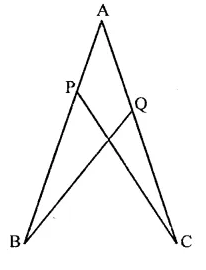Given : In the figure, AB = AC, ∠B = ∠C
To prove : BQ = CP
Proof : In ∆ABQ and ∆ACP
AB = AC (Given)
∠A = ∠A (Common)
∠B = ∠C (Given)
∴ ∆ABQ ≅ ∆ACP (ASA axiom)
∴ BQ = CP (c.p.c.t.)

Todays Deals### RD Chapter 12- Heron-s Formula Ex-VSAQS Contributorskrishan

Name:
Email:

# Latest News# 9000 interview questions in different categories